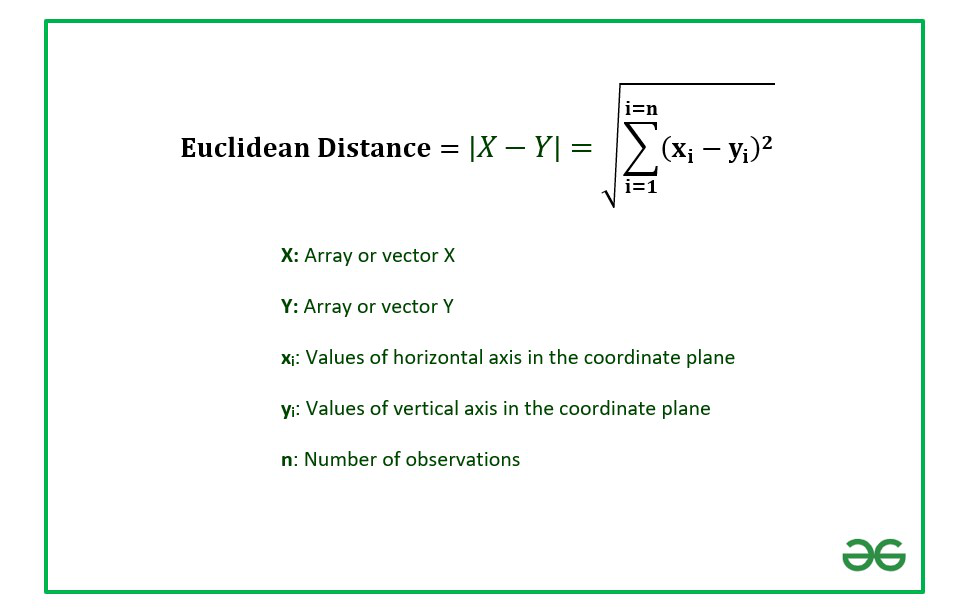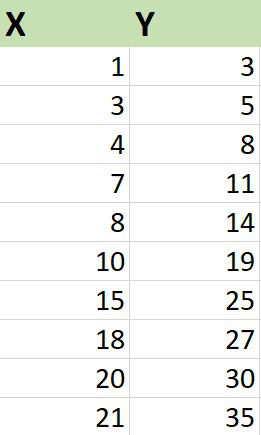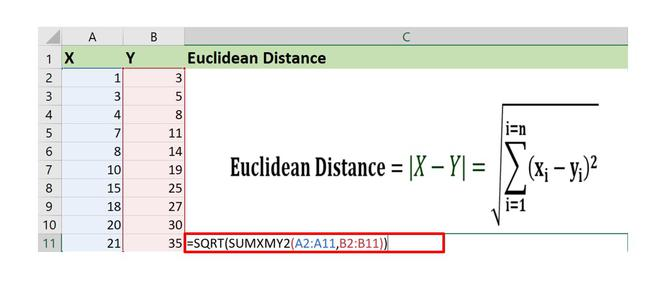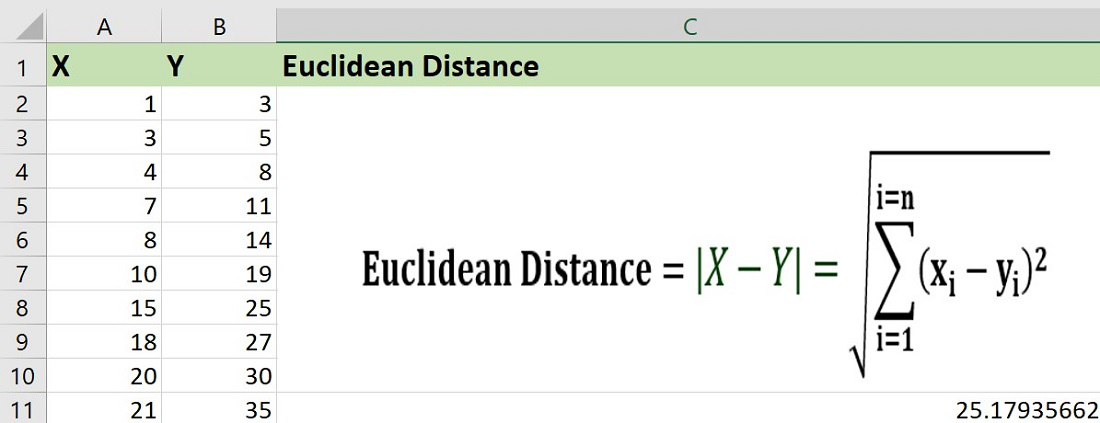# How to Calculate Euclidean Distance in Excel?

• Last Updated : 28 Jul, 2021

Euclidean distance is the distance between two real-valued vectors. It is calculated by the square root of the sum of the squared differences of the elements in the two vectors.

The formula to calculate Euclidean distance is :In this article we are going to discuss how to calculate the Euclidean distance in Excel using a suitable example.

Example : Consider the dataset which consists of information about X and Y coordinates of ten points in a 2-D plane.### Implementation :

The functions used are :

1. SUMXMY2: It finds the sum of squared differences of the elements in array X and array Y.

2. SQRT: It is used to find the square root of the squared difference.

The steps to calculate Euclidean distance are :

1. Insert the coordinates in the Excel sheet as shown above.

Column X consists of the x-axis data points and column Y contains y-axis data points.

2. Write the Excel formula in any one of the cells to calculate the Euclidean distance.

`=SQRT(SUMXMY2(array_x,array_y))`

The observations in array X are stored from A2 to A11.

The observations in array Y are stored from B2 to B11.3. Click on Enter.The Euclidean distance turns out to be 25.18 units approximately.

It is important to note that the number of observations in X and that of Y has to be the same. While calculating the distance Excel takes pairwise values of X and Y simultaneously.

If any one of the values is missing in the worksheet then Excel will ignore that and move to the next data point. So, while entering the dataset we need to be careful to enter the observations X and Y pair-wise such that no data is missing.

My Personal Notes arrow_drop_up